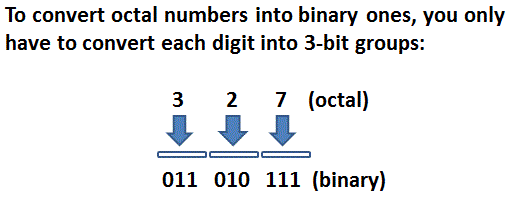# Octal to binary - Two methods in Matlab

To convert a value from octal to binary, we first need to know what an octal number is.

 A major numerical system, or base, used in digital electronics, is the octal system, also known as base 8. In this system of numbers, the symbols are counted only from ‘0’ to ‘7’. In base 8 we use only 8 different symbols. The  binary system (base 2) has only two symbols, '0' and '1'. The following table shows the meaning of all the symbols in the octal system and their conversion to decimal or binary equivalents.

Table equivalents: decimal, octal and binary numbersTo convert a value from octal to binary, you merely translate each octal symbol to the equivalent 3-bit binary group. For example, the octal number 537 translates into the binary 101_011_111 equivalent.

### Solution 1. Conversion from Octal to Decimal, and then Decimal to Binary

In Matlab, we can go from octal to decimal, and then, from decimal to binary. We can use instructions ‘base2dec’ and ‘dec2bin’ for this purpose.

Function base2dec converts base N number string to decimal number. Its syntax is d = base2dec('str', base). For example, base2dec('10', 8) produces an 8.

Function dec2bin converts a decimal number into a binary string. Its syntax is b = dec2bin(d). For example, dec2bin(5) produces the string ‘101’.

We can embed one instruction into the other, like this:

bin_str = dec2bin(base2dec(oct_str, 8))

So, we can use the concept in this way:

oct_str = '537';
bin_str = dec2bin(base2dec(oct_str, 8))

bin_str = 101011111

It’s important to remember that both binary numbers and octal ones are treated as strings in Matlab.

### Solution 2. Conversion to octal using a Switch-Case structure

Now, let’s say that we want to explore how the octal characters are separated to form the binary symbols and how we can manipulate our own strings (binary and octal).We can develop a function to translate the table shown before. Our proposed method uses a switch-case structure.

% Octal to binary conversion
function b = o2b(o)
switch o

case {'0'}
b =
'000';

case {'1'}
b =
'001';

case {'2'}
b =
'010';

case {'3'}
b =
'011';

case {'4'}
b =
'100';

case {'5'}
b =
'101';

case {'6'}
b =
'110';

case {'7'}
b =
'111';
end

Now, we have to call the function for every octal character in the string, to convert each to a 3-bit binary number. One possible solution to separate the octal strings into 3-bit groups is shown here:

oct_str = input('Enter octal number: ', 's');
n = length(oct_str);

bin_str = '';
for o = 1 : n
bin_str = [bin_str o2b(oct_str(o))];

end
bin_str

Let’s try this routine...

Enter octal number: 1515

The result is:
bin_str = 001101001101

Enter octal number: 7070

The result is:
bin_str = 111000111000

From 'Octal to binary' to home

From 'Octal to binary' to 'Matlab Programming'